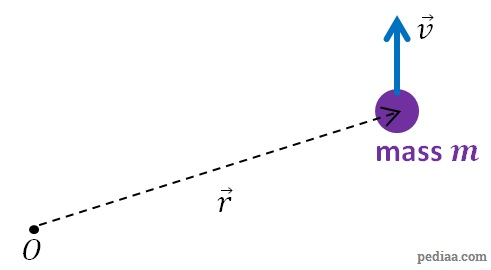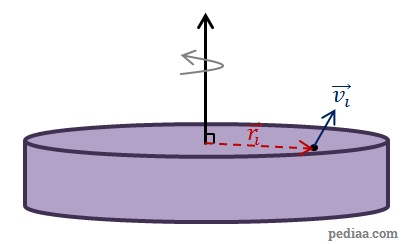# Difference Between Linear Momentum and Angular Momentum

## Main Difference – Linear Momentum vs. Angular Momentum

Momentum is a property of moving objects that have mass. Often, we talk about two types of momenta: linear and angular. The main difference between linear momentum and angular momentum is that linear momentum is a property of an object which is in motion with respect to a reference point (i.e. any object changing its position with respect to the reference point) while angular momentum is a property of objects which are not only changing their position but also the direction of their position with respect to a reference point (i.e. they are not moving in a straight line).

## What is Linear Momentum

Linear momentum of an object is the product of the object’s mass and velocity. Linear momentum is a vector quantity, and the direction of momentum is taken to be the direction of the object’s velocity. If the mass of the object is$m$ and the velocity of the object is$\vec{v}$, then the linear momentum$\vec{p}$ is given by:$\vec{p}=m\vec{v}$

Linear momentum is a conserved quantity: the total linear momentum of particles in a system is conserved if no external forces act on the system. If there is a resultant external force on the system, then the momentum changes, so that the rate of change of momentum is equal to the resultant external force:$\sum \vec{F}=\frac{\mathrm{d}\vec{p}}{\mathrm{d}t}$

The SI units for measuring linear momentum is kg m s-1. We have discussed linear momentum at length in this article.

## What is Angular Momentum

For an object with mass$m$ moving at a velocity$\vec{v}$, the angular momentum$\vec{L}$ with respect to a reference point is defined using the cross product as:$\vec{L}=\vec{r}\times m\vec{v}=\vec{r}\times \vec{p}$

where$\vec{r}$ is the position vector of the object that describes the object’s position with respect to the reference point. The units for measuring angular momentum is kg m2 s-1. Since angular momentum is defined in terms of a cross product, the direction of the angular momentum vector is taken to be in a direction perpendicular to both the particle’s position vector$\vec{r}$ and its velocity vector$\vec{v}$.Defining angular momentum

Using the definition above, we can come up with an expression for calculating angular velocity of a rigid body which is rotating about an axis that is at right angles to the plane in which the particles are rotating. The rigid body is made of many particles, and the sum of angular momenta of all the particles gives the total angular momentum of the rigid body. Then, in terms of the masses and velocities of individual particles, we can write the total angular momentum as:$L=\sum_{i} r_im_iv_i$Finding the angular momentum of a rigid body

Note that since the axis of rotation is perpendicular to the plane in which the particles are rotating, the cross product boils down to a simple multiplication. We can write the linear velocity$v$ of the particles in terms of their angular velocities$\left( \omega\right)$:$L=\sum_{i} {r_i}^2m_i{\omega}_i$

Since the object is rigid, all particles rotate in unison. This means that the angular velocity for all particles is common. Then,$L=\sum_{i}\left( {r_i}^2m_i\right){\omega}$

The quantity$L=\sum_{i}\left( {r_i}^2m_i\right)$ is the object’s  ,$I$. Then, we can write the angular momentum in the object as:$L=I\omega$

Like linear momentum, angular momentum is also a conserved quantity. The angular momentum of a system of particles is conserved if no external torques act on the system. If there is a resultant external torque, the angular momentum changes so that the resultant torque is equal to the rate of change of angular momentum of the object:$\sum \vec{\tau}=\frac{\mathrm{d}\vec{L}}{\mathrm{d}t}$

## Difference Between Linear Momentum and Angular Momentum

### Type of Motion

Linear momentum is a property of objects which are changing their position with respect to a reference point.

Angular momentum is a property of objects which are changing the angle of their position vector with respect to a reference point.

### Conservation

Linear momentum of a system of particles is conserved so long as there is no resultant force on the system.

Angular momentum of a system of particles is conserved so long as there is no resultant torque on the system.

### Rate of Change

The rate of change of linear momentum of a system of particles is equal to the resultant force acting on the system.

The rate of change of angular momentum of a system of particles is equal to the resultant torque acting on the system.

### SI Units

Linear Momentum is measured in units of kg m2 s-1.

Angular momentum is measured in units of kg m2 s-1.